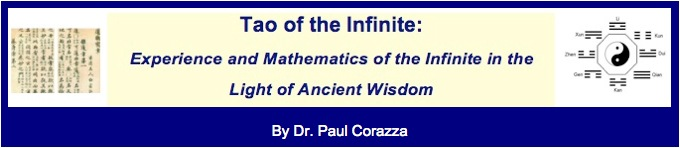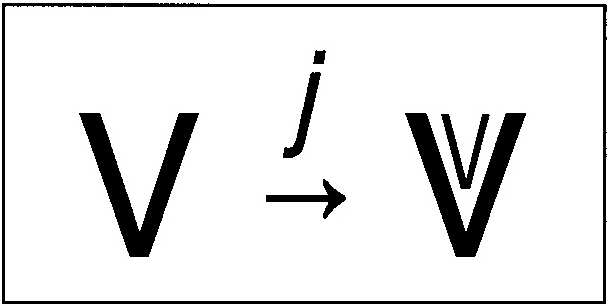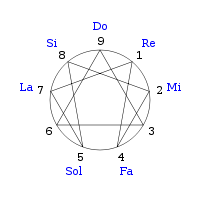Volume 2: The Ancient Wisdom of the Infinite As a Key to Unlock the Mathematics of the Infinite

In Volume 2, Dr. Wu discusses a well-known mathematical problem in set theory, known as the Problem of Large Cardinals. Large cardinals are special types of mathematical infinity that have such strong characteristics, and are so "large," that it is impossible, on the basis of the standard axioms of set theory, to derive their existence; yet, they have not, since their discovery, ever been shown to be inconsistent with the axioms, and in fact, they seem to play a central role in the solution of a number of important problems in many areas of mathematics. The Problem of Large Cardinals is to provide an acceptable solution to this mystery of large cardinals--to provide an account for their origin and presence in the universe by expanding the axioms of set theory (which are in fact the axioms at the basis of all mathematics) in a natural and appropriate way. Dr. Wu elaborates in this volume a unique approach to the problem, which is to broaden the axioms of set theory by making use of principles about the nature of the Infinite as described in the ancient texts. An outline of this program is:
1. Which principles and characteristics of the Infinite as described by the ancients are missing from V?
2. How can these missing values be formulated as a new, mathematically precise axiom, which can be added to the current list of foundational axioms?
3. What can be said about the Problem of Large Cardinals on the basis of this new, expanded foundation for set theory?
4. What other mathematical consequences can be derived from this new axiom system?
Dr. Wu then introduces to the reader the Wholeness Axiom as an answer to these questions--an axiom that has appeared in recent research journals. The Wholeness Axiom is a mathematical formulation of the ancient vision that wholeness, totality, Tao, by its nature, moves within itself, knows itself, and gives rise to all things by way of certain dynamics that unfold within itself.Dr. Wu, drawing upon this recent research, shows how this axiom accounts for virtually all large cardinals and also shows how it introduces into the universe the missing properties and dynamics of the Infinite, as discussed in Volume 1. He also discusses how the new qualities and dynamics introduced into the mathematical universe by this new axiom correspond to qualities and dynamics of the deep nature of one's own consciousness--qualities and dynamics that lead to a profound awakening in human life.

 In the final section of Volume 2, Dr. Wu brings us back to the simplest of all mathematical notions: the natural numbers 1, 2, 3,. . . . As the famous mathematician Leopold Kronecker once put it, "God made the integers. The rest is the work of man." The ancients believed that, in a sense, "everything" comes from the natural numbers. Dr. Wu explores these views and their connection to the subjective experience of the Infinite. Dr. Wu addresses the natural question:Can the dynamics of V, which are given to us by the Wholeness Axiom, be somehow located in seed form in the generation of the natural numbers? The volume is dedicated to explaining why this is indeed the case. This investigation reveals how the basic form of the Wholeness Axiom is already present in a mathematical formulation known to be equivalent to the very existence of the set of natural numbers.

The volume develops this theme, showing how this formulation can be gradually refined to account for larger and larger cardinals, culminating in the Wholeness Axiom. The fact that these profound dynamics of consciousness can be found "so close to home"--in the natural numbers themselves--expresses, as Dr. Wu suggests, that, in reality, the deep profundity of wholeness of the universe is available on a personal level, more intimate even than our own thoughts and feelings.